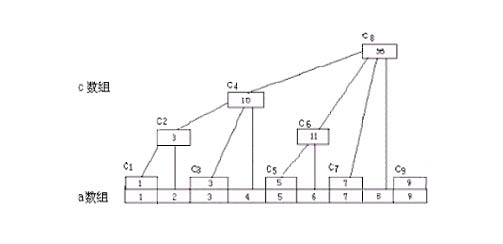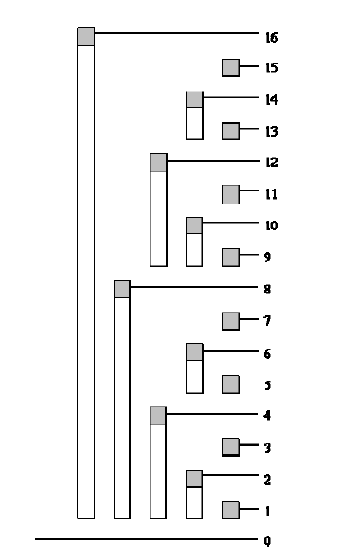## 二、树状数组怎么干的？

### A 图### B 图void add(int k,int num)
{
while(k<=n)
{
tree[k]+=num;
k+=k&-k;
}
}


int read(int k)//1~k 的区间和
{
int sum=0;
while(k)
{
sum+=tree[k];
k-=k&-k;
}
return sum;
}


## 三、总结一下吧

### 1 条评论